# plotting 7 points on a surface so that

Hi, sorry if I can't explain you the question properly. We need to plot 7 point on a surface so that: after picking any 3 point, the distance between at least two points should be exactly 1 unit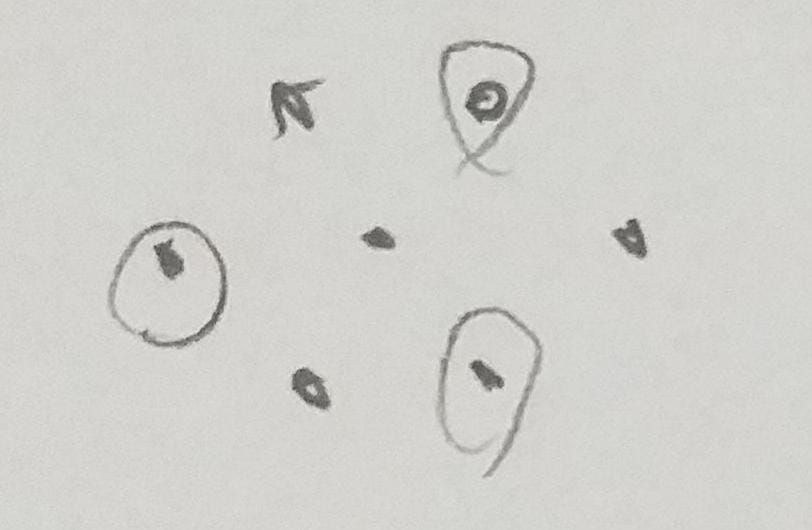for e.g I thought hexagon and a point in the center. But it does not be true for every 3 points. I can't find solution more than 6 points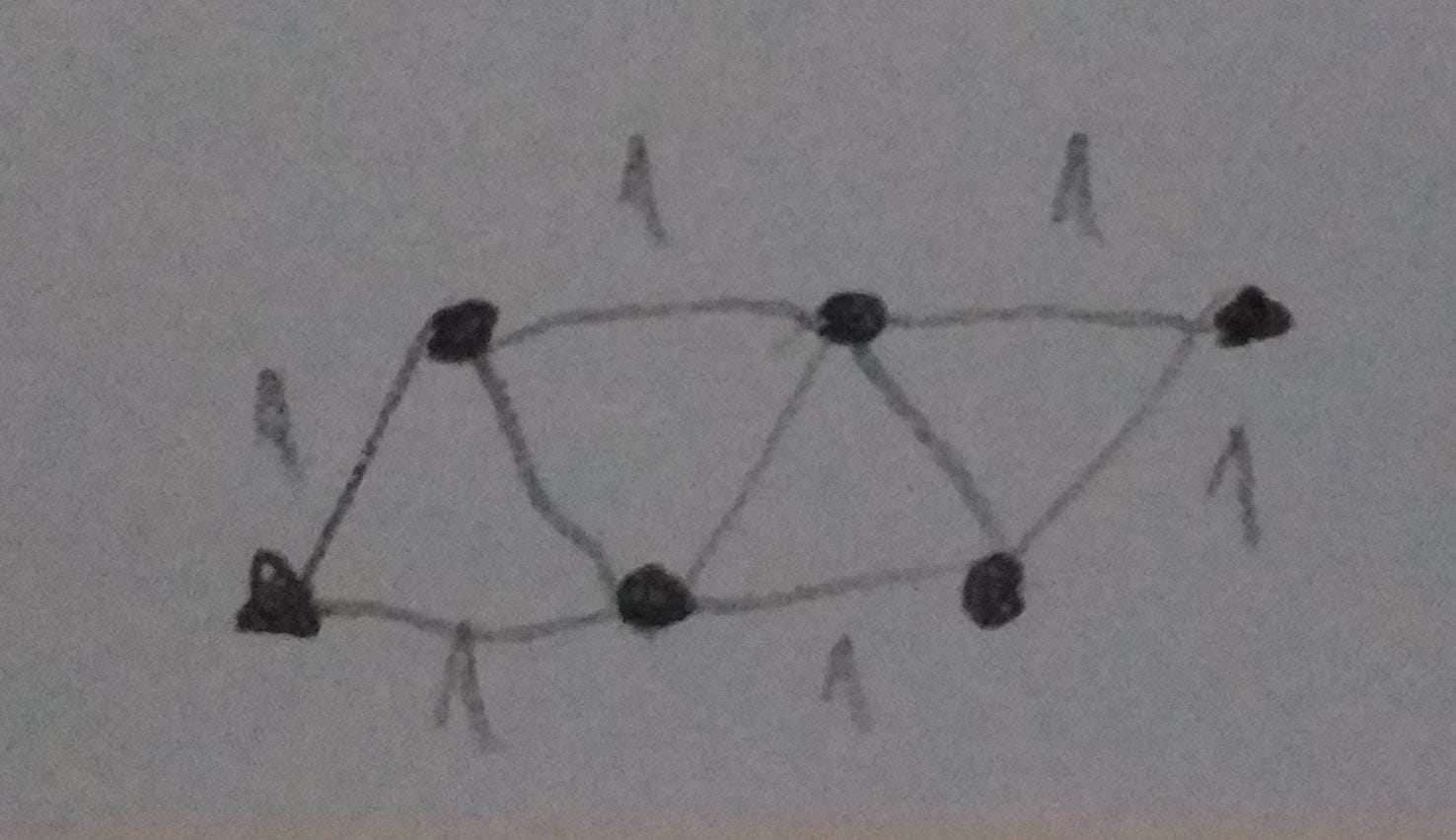Note by Emre Tuzcu
4 months ago

This discussion board is a place to discuss our Daily Challenges and the math and science related to those challenges. Explanations are more than just a solution — they should explain the steps and thinking strategies that you used to obtain the solution. Comments should further the discussion of math and science.

When posting on Brilliant:

• Use the emojis to react to an explanation, whether you're congratulating a job well done , or just really confused .
• Ask specific questions about the challenge or the steps in somebody's explanation. Well-posed questions can add a lot to the discussion, but posting "I don't understand!" doesn't help anyone.
• Try to contribute something new to the discussion, whether it is an extension, generalization or other idea related to the challenge.

MarkdownAppears as
*italics* or _italics_ italics
**bold** or __bold__ bold
- bulleted- list
• bulleted
• list
1. numbered2. list
1. numbered
2. list
Note: you must add a full line of space before and after lists for them to show up correctly
paragraph 1paragraph 2

paragraph 1

paragraph 2

[example link](https://brilliant.org)example link
> This is a quote
This is a quote
    # I indented these lines
# 4 spaces, and now they show
# up as a code block.

print "hello world"
# I indented these lines
# 4 spaces, and now they show
# up as a code block.

print "hello world"
MathAppears as
Remember to wrap math in $$ ... $$ or $ ... $ to ensure proper formatting.
2 \times 3 $2 \times 3$
2^{34} $2^{34}$
a_{i-1} $a_{i-1}$
\frac{2}{3} $\frac{2}{3}$
\sqrt{2} $\sqrt{2}$
\sum_{i=1}^3 $\sum_{i=1}^3$
\sin \theta $\sin \theta$
\boxed{123} $\boxed{123}$

Sort by: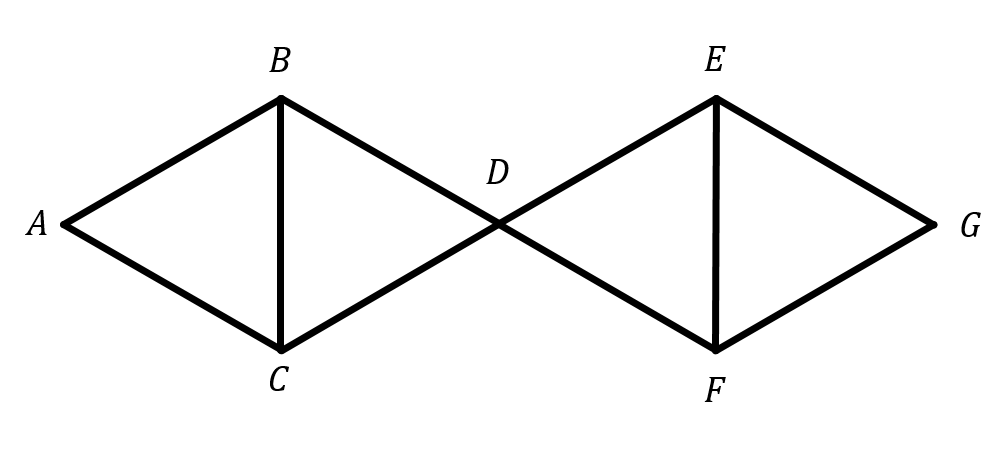You can see this works in every case except, when we choose A,D and G. Now if we rotate the right side in this way $\overline{AG}=1$ then we solved the problem: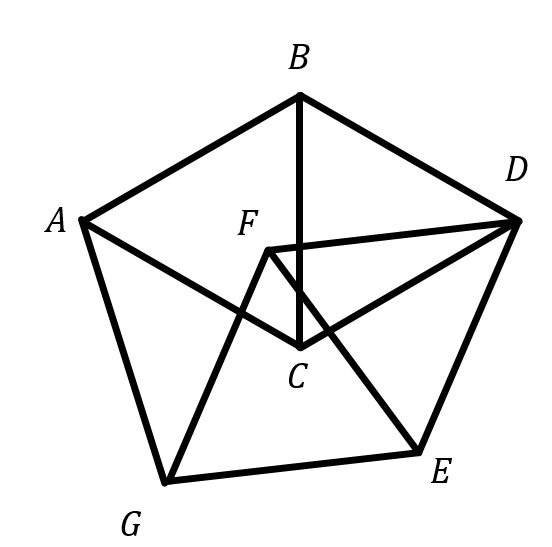Actually this looks logical but is it true for BFG

- 4 months ago

- 4 months ago

Right fool me :)

- 4 months ago

What does BFG mean? I found only Big F$***$ing Gun

That was right. I thought wrong for a sec

- 4 months ago

$\leq1$ or exactly one unit?

Exactly one unit. Also I think there may not be a solution

- 4 months ago

If exactly one this is impossible. If you draw an equilateral triangle and write the circles with radius=1, than there won't be engraving of the three lones in one point, so the number of points should be less than 4

Maybe in higher dimensions

Thanks for reply. Can you explain your proof withmore details. I didn't get it It may be impossible for 7 points but not for 5 or 6 points. If you draw a hexagon, it satisfies the conditions. I think it's impossible for >=7 points

- 4 months ago

If the task says that the distance between all of the points should be 1 unit, then the biggest number of points is 3. Only the mentioned triangle can satisfy the conditions. But if the task says that we can choose two from the three points, then the hexagon won't work. If the name of the hexagon is ABCDEF, than the distance between A, C and E isn't one unit.

That's right but its true for square or pentagon

- 4 months ago

Yeah. Here is an interesting problem: Given 2006 points. In every case when we choose 3 points, then the area of the triangle with the selected points won't be bigger than 1 unit. Prove that we can cover these points with a triangle with 4 units area!

From XV. NNMV 9. class.

okay but actually this is totally different question

- 4 months ago

anyway, thanks for you attention

- 4 months ago

You are wolcome!

*welcome

I have a figure with 6 points which satisfies the condition mentioned in the notes. I can share it if you want

- 4 months ago

I doubt it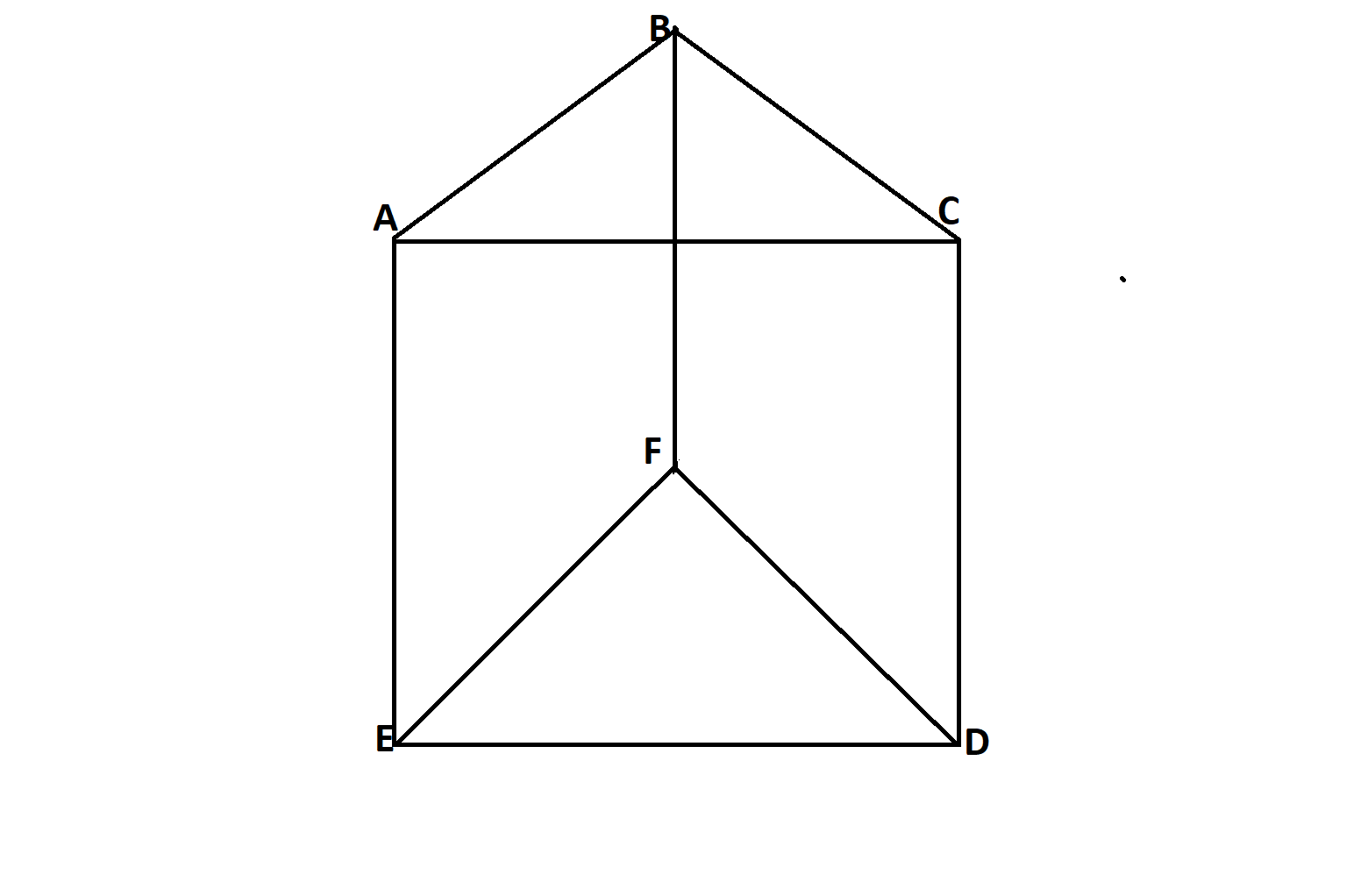In the above diagram $ACDE$ is a square with side of one unit length. $\triangle ABC$ and $\triangle FED$ are equilateral.

Now in parallelogram $ABFE$, $\overline{FB}$ is also of 1 unit length.

- 4 months ago

I tried to show it with dots on the keyboard but the blanks does not count here. If you think 2 equilateral triangle touching each other at the bottom (one's right bottom corner touches the others left bottom corner) This makes 5 point and 2 on the upside and 3 on the bottom, so draw last point to make 2 upside down triangle on the upside

- 4 months ago

I don't understand what does it mean :( The maximum number of dots is 5.

I edited it to the question

- 4 months ago

Nice work! I will write a c++ program when I have time.

I solved it with 7 points

Maybe incorrect, but I'm testing it yet

Thank you so much for your attention, now I can sleep in peace :) @Pàll Màrton and @Sahar Bano

- 4 months ago

You posted it 3 days ago :)

it was rough time :)

- 4 months ago

No! The rough time, when you must eat the food at school.

Ahahah Actually I eat at the college no problem every time I go to school. Why its bothering you

- 4 months ago

The school has no money. That's something it looks like:oh, dude. I get it now. We usually eat better than this

- 4 months ago

also, enjoy your meal. If you can :)

- 4 months ago